## 2012年1月26日星期四

### HKSAR CE Candidates' Capability - Data Analysis (以品質管理原則看特首候選人能力的調查(數據分析))

When the survey period closed, all data were validated and analyzed. Firstly, Normality Test was performed to examine whether or not the observations followed a normal distribution. The hypotheses were shown as follows:

H0: data follow a normal distribution vs. H1: data do not follow a normal distribution

If Normality Test passes, parametric hypothesis test (2 sample t-test) would be employed to compare each candidate's capability (mean value) with 95% confidence level. If Normality Test fail, non-parametric hypothesis test (Mann-Whitney test) would be used to compare each candidate's capability (median value) with 95% confidence level.

One of the simplest versions of the Central Limit Theorem (CLT) says that if it is a random sample of size n (say, n> 30) from an infinite population finite standard deviation, then the standardized sample mean converges to a standard normal distribution or, equivalently, the sample mean approaches a normal distribution with mean equal to the population mean and standard deviation equal to standard deviation of the population divided by square root of sample size n.

73 independent responded data set were collected and CLT could be used with assumption of a normal distribution. However, all data failed the Normality Test. It may be the result of using seven-point likert scale in which each data point was an integral. Therefore, both parametric and nonparametric hypothesis test were used to exam the data sets. If same conclusion is obtained from both hypothesis tests, it would be taken as final result.

The codes used in this analysis were given again.

HKSAR Chief Executive Candidates 2012 on 1st Jan 2012 were:

- Mr. Henry TANG Ying-yen (唐英年) (HT)

- Mr. LEUNG Chun-ying (梁振英) (CY)

- Mr. Albert HO Chun-yan (何俊仁) (AH)

- Mr. Frederick FUNG Kin-kee (馮檢基) (FF)

The 8 questions using the Eight Quality Principles (code) were shown below:

Seven-point likert scale used as follows:

(Capability Level: 7－Very Capable，６－Comparative Capable，５－ Slightly Capable，４－Accepted Capable，３－Slightly Incapable，２－Comparative Incapable，１－Very Incapable)

(能力水平：7－非常有能力，６－比較有能力，５－稍多的能力，４－可接受的能力，３－稍遜的能力，２－比較沒能力，１－非常沒能力)

Hypothesis test presentation:

"»" is statistical significant that p ~ 0.000

">" is statistical significant that p is below 0.05

"~>" is not statistical significant but p is around 0.1

"~" is not statistical significant

Data Analysis

Data distribution of each candidate in normality test:Boxplot (Summary) for Customer Focus of each candidate: (The circle (+) is mean and (x) is median.)

Hypothesis test I (for different - two tailed test):

Hypothesis test II (for greater - one tailed test):

Conclusion:

It found that CY had much higher score than other candidates in the Customer/Citizen Focus which was statistically significant for 95% confident level.

CY » HT ~ AH ~ FF

Data distribution of each candidate in normality test:Boxplot (Summary) for Leadership of each candidate: (The circle (+) is mean and (x) is median.)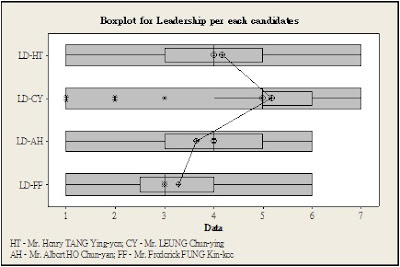Hypothesis test I (for different - two tailed test):

Hypothesis test II (for greater - one tailed test):

Conclusion:

It found that CY had much higher score than other candidates in the Leadership which was statistical significant for 95% confident level; and HT had much higher score than the other two candidates. AH was found statistically significant and had higher score than FF.

CY » HT » AH > FF

Data distribution of each candidate in normality test:Boxplot (Summary) for Involvement of People of each candidate: (The circle (+) is mean and (x) is median.)

Hypothesis test I (for different - two tailed test):

Hypothesis test II (for greater - one tailed test):

Conclusion:

It found that CY had much higher score than other candidates in the Involvement of People which was statistically significant for 95% confident level.

CY » HT ~ AH ~ FF

Data distribution of each candidate in normality test: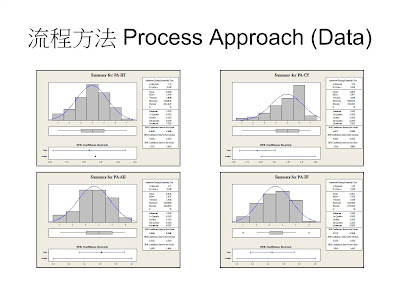Boxplot (Summary) for Process Approach of each candidate: (The circle (+) is mean and (x) is median.)

Hypothesis test I (for different - two tailed test):

Hypothesis test II (for greater - one tailed test):

Conclusion:

It found that CY had much higher score than other candidates in the Process Approach which was statistically significant for 95% confident level. HT had higher score than FF with statistically significant for 95% confident level.

CY » HT / AH / FF

HT > FF

Data distribution of each candidate in normality test:Boxplot (Summary) for System Approach to Management of each candidate: (The circle (+) is mean and (x) is median.)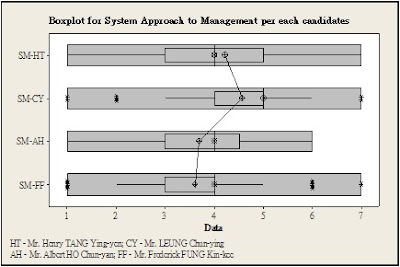Hypothesis test I (for different - two tailed test):Hypothesis test II (for greater - one tailed test):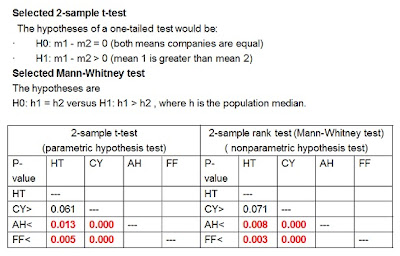Conclusion:

It found that CY and HT had much higher score than other candidates in the System Approach to Management which was statistically significant for 95% confident level. CY had slight higher score than HT which was statistically significant for 90% confident level.

CY ~> HT » AH ~ FF

Data distribution of each candidate in normality test: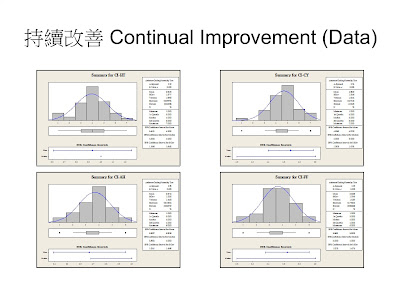Boxplot (Summary) for Continual Improvement of each candidate: (The circle (+) is mean and (x) is median.)

Hypothesis test II (for greater - one tailed test):

Conclusion:

It found that CY had much higher score than other candidates in the Continual Improvement which was statistically significant for 95% confident level. HT had higher score than AH and FF which was statistically significant for 90% confident level.

CY » HT ~> AH ~ FF

Data distribution of each candidate in normality test:Boxplot (Summary) for Factual Approach to Decision Making of each candidate: (The circle (+) is mean and (x) is median.)

Hypothesis test I (for different - two tailed test):

Hypothesis test II (for greater - one tailed test):

Conclusion:

It found that CY had much higher score than other candidates in the Factual Approach to Decision Making which was statistically significant for 95% confident level.

CY » HT ~ AH ~ FF

Data distribution of each candidate in normality test:Boxplot (Summary) for Mutually Beneficial Supplier Relationships of each candidate: (The circle (+) is mean and (x) is median.)

Hypothesis test II (for greater - one tailed test):

Conclusion:

It found that CY and HT had much higher score than other candidates in the Mutually Beneficial Supplier Relationships which was statistically significant for 95% confident level.

CY ~ HT » AH ~ FF

The overall conclusion and consolidated summary would be issued.

Reference:

John McKenzie, Robert L.Schaefer & Elizabeth Farber (1995) "The Student Edition of MINITAB for Windows - Statistical software ... adapted for education" Addison-Wesley Publishing Company, Inc.